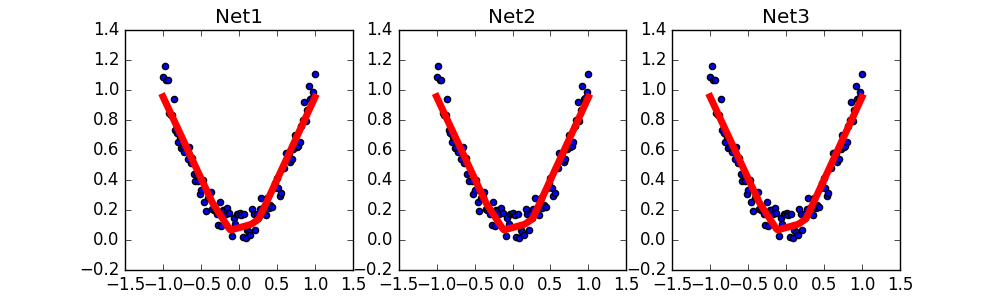# 保存提取

## 保存 ¶

torch.manual_seed(1)    # reproducible

# 假数据
x = torch.unsqueeze(torch.linspace(-1, 1, 100), dim=1)  # x data (tensor), shape=(100, 1)
y = x.pow(2) + 0.2*torch.rand(x.size())  # noisy y data (tensor), shape=(100, 1)

def save():
# 建网络
net1 = torch.nn.Sequential(
torch.nn.Linear(1, 10),
torch.nn.ReLU(),
torch.nn.Linear(10, 1)
)
optimizer = torch.optim.SGD(net1.parameters(), lr=0.5)
loss_func = torch.nn.MSELoss()

# 训练
for t in range(100):
prediction = net1(x)
loss = loss_func(prediction, y)
loss.backward()
optimizer.step()


torch.save(net1, 'net.pkl')  # 保存整个网络
torch.save(net1.state_dict(), 'net_params.pkl')   # 只保存网络中的参数 (速度快, 占内存少)


## 提取网络 ¶

def restore_net():
# restore entire net1 to net2
prediction = net2(x)


## 只提取网络参数 ¶

def restore_params():
# 新建 net3
net3 = torch.nn.Sequential(
torch.nn.Linear(1, 10),
torch.nn.ReLU(),
torch.nn.Linear(10, 1)
)

# 将保存的参数复制到 net3
prediction = net3(x)


## 显示结果 ¶

# 保存 net1 (1. 整个网络, 2. 只有参数)
save()

# 提取整个网络
restore_net()

# 提取网络参数, 复制到新网络
restore_params()